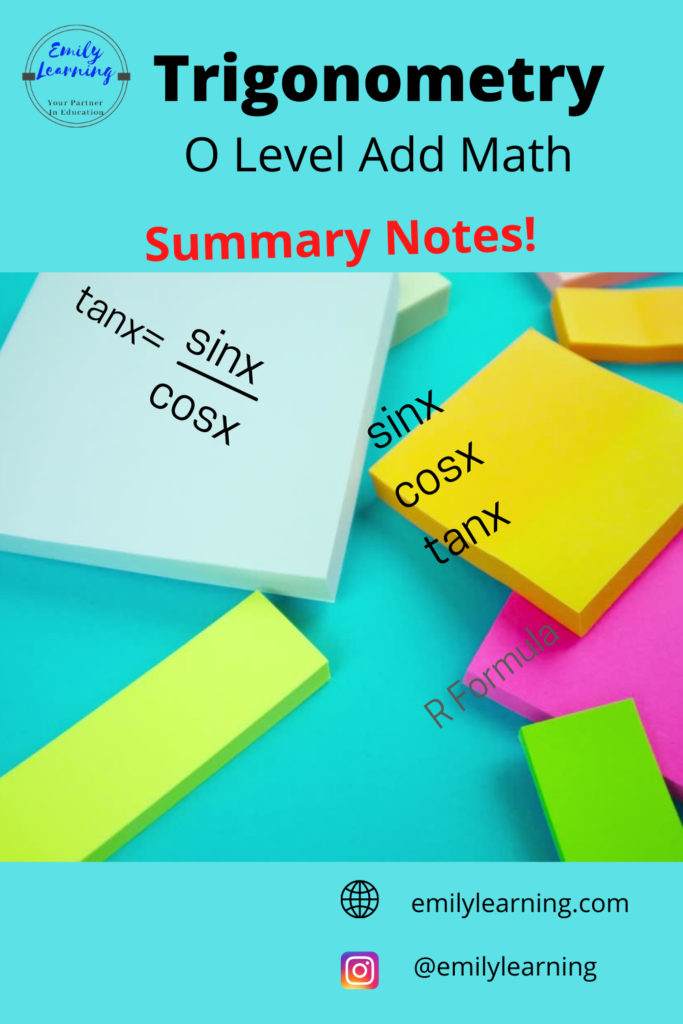# Summary Notes for Trigonometry – O Level Add MathHere’s a summary of Trigonometry for O Level Additional Mathematics. I go through what’s tested in this  article here

## Assumed Trigonometry Knowledge for O Level Add Math:

For right angle triangles:

• sin x = opposite/ hypotenuse
• cos x = adjacent/ hypotenuse
• tan x = opposite/ adjacent
• Pythagoras Theorem: (hypotenuse)2 = (opposite)2 + (adjacent)2

For non- right angle triangles, you will use sine rule and cosine rule. These are found in the formula booklet of your exam, so don’t memorize them (just know where they are).

• sine rule:
• a/sin∠A = b/sin∠B = c/sin∠C
• cosine rule:
• c2 = a2+ b– 2abcos∠C

## Trigonometric Ratios

These are some trigonometric ratios that you are expected to know (note: they are not in you data booklet, so make sure you know them!)
cosec x  = 1/ sin x
sec x = 1/ cos x
cot x = 1/ cot x
tan x = sinx / cosx

# Principal Value of inverse functions

-90 o ≤ sin -1x ≤ 90 o
-90 o ≤ tan -1x ≤ 90 oo ≤ cos -1≤ 180 o

## Range of values of cos and sin

-1 ≤ cos x ≤ 1-1 ≤ sin x ≤ 1

## Amplitude and period of graphs

For y = a cos(bx + c) + d or y = a sin (bx + c) + d
Period  = 360 o/bAmplitude = |a|maximum value = |a| +dminimum value = – |a| + d
For y = a tan(bx+c)Period  = 180 o/b

## Graph of  y  = tan x

To solve trigonometry equations, you need to know when sin/ cos/ tan are positive (or negative). Remember this quadrant, or the A-S-T-C in short.

## Trigonometric Identities

You use trigonometric identities for solving and proofing identity questions. These identities are inside your formula booklet, you don’t need to memorize them. Just know that they are there.

## R- Formula

This is not in your formula booklet. Make sure you know them:
a sin x ± b cos x = R cos (x ± α) a cos x ± b sin x = R cos (x ∓ α)
R = square root(a2 + b2)α = tan-1 (b/a)

## Want to learn O Level Add Maths on-demand?

Want to learn O Level Add Math on-demand? Check out our course here.

error: Content is protected !!